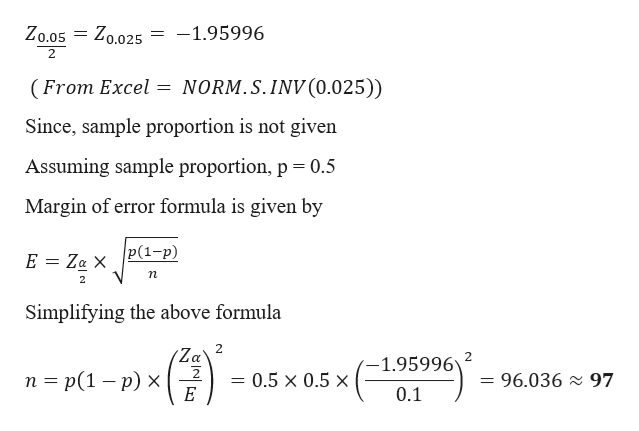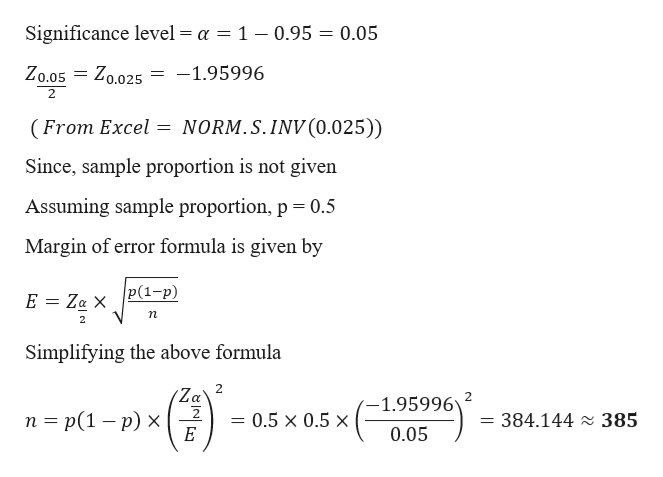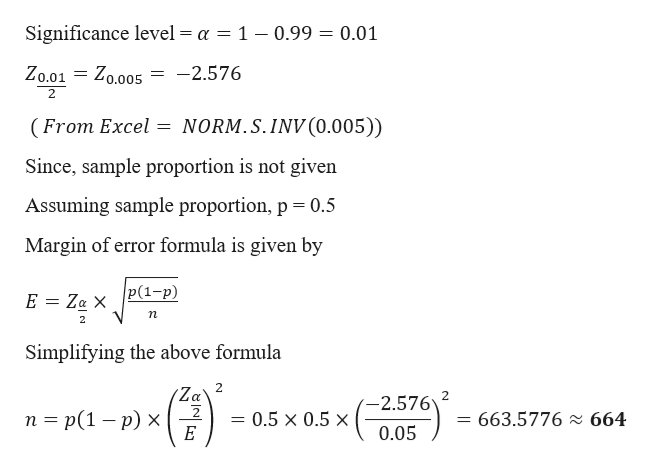Question
51 views

8.50 A,B,C

check_circle

Step 1

a)

Given data

Error =  0.10

Confidence level = 0.95help_outlineImage TranscriptioncloseZo.05 Zo.025 -1.95996 2 NORM.S.INV (0.025)) (From Excel = Since, sample proportion is not given Assuming sample proportion, p 0.5 Margin of error formula is given by |Р(1-р) E Za X п 2 Simplifying the above formula 2 Za 1.95996 п %3 p(1 — р) х = 96.036 97 0.5 x 0.5 x E 0.1 fullscreen
Step 2

b)

Given data

Error =  0.05

Confidence level = 0.95help_outlineImage TranscriptioncloseSignificance level = a = 1 - 0.95 = 0.05 = Zo.025 -1.95996 Zo.05 2 NORM.S.INV (0.025)) (From Excel = Since, sample proportion is not given Assuming sample proportion, p 0.5 Margin of error formula is given by |Р(1-р) E Za X п Simplifying the above formula 2 Za 2 п %3 p(1 — р) х 1.95996 0.5 x 0.5 x = 384.144 2 385 0.05 Е fullscreen
Step 3

c)

Given data

Error =  0.10

Confidence ...help_outlineImage TranscriptioncloseSignificance level = a = 1 - 0.99 = 0.01 Zo.01 = Zo.005 -2.576 2 (From Excel NORM.S.INV(0.005)) Since, sample proportion is not given Assuming sample proportion, p 0.5 Margin of error formula is given by P(1-p) E Za X n Simplifying the above formula 2 Za' 2 E -2.576 n p(1 - p) x 663.5776 664 0.5 x 0.5 x 0.05 fullscreen

### Want to see the full answer?

See Solution

#### Want to see this answer and more?

Solutions are written by subject experts who are available 24/7. Questions are typically answered within 1 hour.*

See Solution
*Response times may vary by subject and question.
Tagged in

### Other